# DAV Class 5 Maths Chapter 9 Brain Teasers Solutions

The DAV Maths Book Class 5 Solutions and DAV Class 5 Maths Chapter 9 Brain Teasers Solutions of Rounding Numbers offer comprehensive answers to textbook questions.

## DAV Class 5 Maths Ch 9 Brain Teasers Solutions

Question 1.
(a) 3859 rounded off to the nearest ten is:
(i) 4000
(ii) 3850
(iii) 3860
(iv) 3100
Solution:
(iii) 3860

(b) 3.841 rounded off to the nearest hundredth is:
(i) 3.840
(ii) 3.800
(iii) 3.940
(iv) 3.850
Solution:
(i) 3.840(c) Sum of 13 and 71 rounded off to the nearest ten is:
(i) 85
(ii) 90
(iii) 95
(iv) 80
Solution:
(iv) 80
i.e., 13 + 71 = 84 rounded off 80

(d) 9999 rounded off to the nearest thousand is:
(i) 9000
(ii) 9900
(iii) 10000
(iv) 990
Solution:
(iii) 10,000

(e) While rounding off numbers to the nearest lakh, we look at the digit in the ________ place.
(i) lakhs
(ii) ten thousands
(iii) thousands
(iv) hundreds
Solution:
(ii) ten thousands
i.e., For lakh, we look at digits in ten thousands place.

Question 2.
Round off each of the following numbers to the nearest ten, hundred, and thousand.
(a) 5,836
(b) 9,873
(c) 2,093
Solution: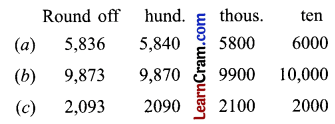Question 3.
Rounded off each of the following numbers to the nearest ten thousand and ten lakh.
(a) 58,58,392
(b) 99,80,565
(c) 44,44,444
Solution:Question 4.
Round off each of the following decimal numbers to the nearest ones, tenth, and hundredth.
(a) 3.081
(b) 18.546
(c) 9.987
Solution: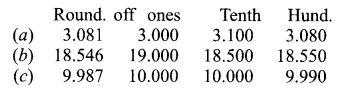Question 5.
The population of some Indian States according to the 2011 census is given below. Complete the table. The first one is done for you.Solution: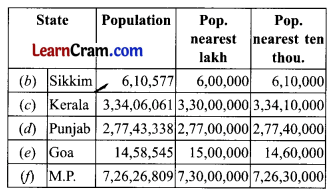Question 6.
Add 571, 285, 199, and round off their sum to the nearest hundred.
Solution:Rounding off 1055 to the nearest hundred = 1,100

Question 7.
Round off the product of 7,48,392 and 296 to the nearest ten crore.
Solution:
The product of numbers is 748392 × 296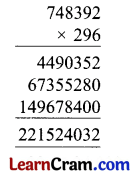Product = 22,15,24,032
Rounding off the product to the nearest ten crore = 20,00,00,000

Question 8.
Divide 60.35 by 25 and round off the quotient to the nearest ones.
Solution: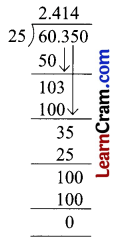Rounding off 2.414 to the nearest ones = 2.000Question 9.
Round off the difference of 13.256 and 7.93 to the nearest hundredth.
Solution:
Difference of numbers = 13.256 – 7.930 = 5.326
Rounding off 5.326 to the nearest hundredth is 5.330.

Question 1.
Round off the following numbers to the nearest ten.
(a) 85
Solution:
85 → 90

(b) 148
Solution:
148 → 150

(c) 1,038
Solution:
1,038 → 1,040

(d) 557
Solution:
557 → 560

Question 2.
Round off the following numbers to the nearest hundred.
(a) 770
Solution:
770 → 800

(b) 1,021
Solution:
1,021 → 1,000(c) 980
Solution:
980 → 1,000

(d) 270
Solution:
270 → 300

Question 3.
Round off the given numbers to the nearest lakh.
(a) 7,50,000
Solution:
7,50,000 → 8,00,000

(b) 9,67,329
Solution:
9,67,329 → 10,00,000

(c) 8,45,132
Solution:
8,45,132 → 8,00,000

Question 4.
Estimate the product of 32 and 68 by rounding off to the nearest hundred.
Solution:Rounding off the product to the nearest hundred.
2,176 → 2,200

Question 5.
Add the following and round off their sum to the nearest thousand.
(a) 1200, 3250, 5620
Solution:10,070 → 10,000
(Round off to the nearest thousand)(b) 7351, 5430, 1331
Solution:14,112 → 14,000
(Round off to the nearest thousand)

(c) 9098, 1240, 1001
Solution:11,339 → 11,000
(Round off to the nearest thousand)

Question 6.
(a) 2537 rounded off to the nearest hundred is:
(i) 2600
(ii) 2540
(iii) 2500
(iv) 2550
Solution:
(iii) 2500

(b) Product of 61 and 33 rounded off to the nearest hundred is:
(i) 2100
(ii) 2000
(iii) 2010
(iv) 2020
Solution:
(ii) 2000(c) 99834 rounded off to the nearest thousand is:
(i) 99000
(ii) 98000
(iii) 10,000
(iv) 1,00,000
Solution:
(iv) 1,00,000